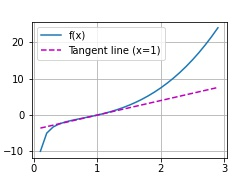# Calculus

#### Exercise 1

The derivative of:

is:

At x = 1 we get y = 0 and a slope for the tangent of 4
The tangent will have an intercept of -4 and an equation of 4x-4

To plot:

def f(x):
return(x**3-1/x)

#div by 0 error at x=0, so we use x=0.1
x = np.arange(0.1, 3, 0.1)
fig = plot(x, [f(x), 4 * x-4], 'x', 'f(x)', legend=['f(x)', 'Tangent line (x=1)'])


The image will be:To save this image add this line to the beginning of the plot function:

    fig = d2l.plt.figure()


    return fig


and this after making the plot:

fig.savefig("2_Prelim 4_Calc 1_Ex.jpg")

1 Like

### Exercise 2

1 Like

I appreciate any corrections!

### Exercise 3

Explaination:

use the chain rule:

### Exercise 4

1 Like

Your solution is so brief and correct. Thank you